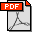J. Gao, L. J. Guibas, A. Nguyen, Deformable Spanners and Applications, Computational Geometry: Theory and Applications, 35:2-19, 2006. Abstract: For a set \$S\$ of points in \$\reals^d\$, an \$s\$-spanner is a graph on \$S\$ such that any pair of points is connected via some path in the spanner whose total length is at most \$s\$ times the Euclidean distance between the points. In this paper we propose a new sparse \$(1+\eps)\$-spanner with \$O(n/\eps^d)\$ edges, where \$\eps\$ is a specified parameter. The key property of this spanner is that it can be efficiently maintained under dynamic insertion or deletion of points, as well as under continuous motion of the points in both the kinetic data structures setting and in the more realistic blackbox displacement model we introduce. Our deformable spanner succinctly encodes all proximity information in a deforming point cloud, giving us efficient kinetic algorithms for problems such as the closest pair, the near neighbors of all points, approximate nearest neighbor search (aka approximate Voronoi diagram), well-separated pair decomposition, and approximate \$k\$-centers. Bibtex: ```@article{agnrz-cddn-04, , author = "Jie Gao and Leonidas Guibas and An Nguyen" , journal = "Computational Geometry: Theory and Applications" , title = "Deformable Spanners and their Applications" , year = 2006 , volume = 35 , pages = "2-19" }```